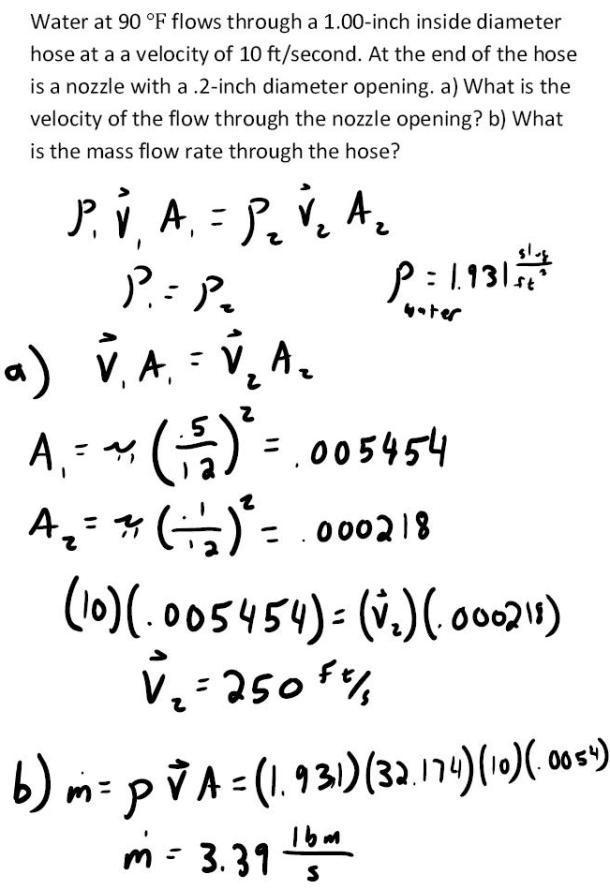fluid mechanics math problems engineering equations fluid mechanics basic mechanics school homework engineering math fluids formulas fluid mechanics problems fluid mechanics problem solutions to fluids problems full solution fluid mechanics engineering fluids problem solution fluid mechanics math problems engineering equations fluid mechanics basic mechanics school homework engineering math fluid mechanics formulas fluid problems fluid mechanics problem solutions to fluid mechanics problems full solution fluid mechanics engineering fluid mechanics problem solution
fluid mechanics math problems engineering equations fluid mechanics basic mechanics school homework engineering math fluids formulas fluid mechanics problems fluid mechanics problem solutions to fluids problems full solution fluid mechanics engineering fluids problem solution fluid mechanics math problems engineering equations fluid mechanics basic mechanics school homework engineering math fluid mechanics formulas fluid problems fluid mechanics problem solutions to fluid mechanics problems full solution fluid mechanics engineering fluid mechanics problem solution
Highalphabet Home Page fluid mechanics solutions fluids math solved Fluid Mechanics Page 1
Water at 90 degrees F flows through a 1.00 inch inside diameter hose at a velocity of 10 ft/second. At the end of the hose is a nozzle with a .2 inch diameter opening. a) What is the velocity of the flow through the nozzle opening? b) What is the mass flow rate through the hose?Water at 90 degrees F flows through a 1.00 inch inside diameter hose at a velocity of 10 ft/second. At the end of the hose is a nozzle with a .2 inch diameter opening. a) What is the velocity of the flow through the nozzle opening? b) What is the mass flow rate through the hose?